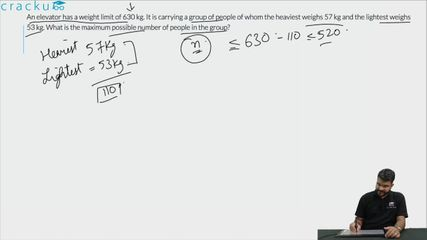Question 69

# An elevator has a weight limit of 630 kg. It is carrying a group of people of whom the heaviest weighs 57 kg and the lightest weighs 53 kg. What is the maximum possible number of people in the group?

Solution

It is given that the maximum weight limit is 630. The lightest person's weight is 53 Kg and the heaviest person's weight is 57 Kg.

In order to have maximum people in the lift, all the remaining people should be of the lightest weight possible, which is 53 Kg.

Let there be n people.

53 + n(53) + 57 = 630

n is approximately equal to 9.8. Hence, 9 people are possible.

Therefore, a total of 9 + 2 = 11 people can use the elevator.

### View Video Solution• All Quant CAT Formulas and shortcuts PDF
• 30+ CAT previous papers with solutions PDF

##### Anjali Chindalia

2 months ago

Hello sir,
I have calculated this ques like:
630÷53= 11.88
630÷ 57= 11.05
Round off- 11 people
Is this the right way of doing the ques?